# Quadray Coordinates: A Python Implementation

by Kirby Urner
First posted: May 29, 2000
Last updated: May 29, 2000

BACKGROUND

Quadrays may be considered a subclass of a more generic Vector class. Quadrays define four basic directions, from the center of a regular tetrahedron to its four corners, and map all other points in space relative to these four vectors, labeled (1,0,0,0), (0,1,0,0), (0,0,1,0), (0,0,0,1) -- with (0,0,0,0) their common origin.

Left and right handed versions may be distinguished according to whether (1,0,0,0), (0,1,0,0), (0,0,1,0) appear in a clockwise or counterclockwise order, looking from the viewpoint of the fourth vertex (0,0,0,1).

Are quadrays new? They certainly have some features in common with the barycentrics and other homogenous coordinate systems. This essay summarizes the work of various individuals without advancing anyone's claims to priority. I invite readers to provide me with feedback about prior and/or contemporaneous partially overlapping research.

GEOMETRIC IMPLEMENTATION

Quadrays may be scaled and added in the usual fashion when considered geometrically, i.e. a vector sum may be depicted as a series of arrows placed tip to tail. The end result is a vector from the origin to the last vector tip. Multiplication by a floating point or real number k stretches a "Qvector" if k>1 and shrinks it if 0=<k<1. If k<0, the negative sign behaves as a "reversal operator," reorienting a Qvector by 180 degrees, after which it is scaled by |k|. These geometric cartoons are essentially equivalent to the Cartesian ones.

ALGEBRAIC IMPLEMENTATION

Algebraically, the methods are slightly different. No vector need involve all four quadrays in its construction, as any point is either:

• in one of four quadrants
• in a plane between Qvectors
• on a basis Qvector
• is the origin itself

The corresponding 4-tuples will have 1,2,3 and 4 zeros respectively, i.e. at least one coordinate will always be 0. We may write this as {a,b,c,0} for a,b,c >=0, where {} means "all permutations of the enclosed members". Note also that we have no need for negative numbers in the normalized 4-tuple, given all four basis vectors are positive (or unsigned).

COMPUTER LANGUAGE IMPLEMENTATION

My background as a math teacher, computer literarcy text book contributor, and computer programmer, predisposes me to blend more conventional mathematics notations with a programming language.

In March, 1999, FoxPro Advisor, a trade magazine for Xbase application developers, published my article about using quadrays to drive ray tracings of colorful polyhedra.

Since writing that article, I've become enamored of Python as a teaching language, because of the interactive command line environment and spare, readable syntax. And unlike Xbase, Python is free and cross-platform.

The section below is a transcript of an interactive Python session, with >>> prompting user input. Computer output is in blue, and flush to the left.

``` Python 1.6a2 (#0, Apr  6 2000, 11:45:12) [MSC 32 bit (Intel)] on win32
Copyright 1991-1995 Stichting Mathematisch Centrum, Amsterdam
IDLE 0.6 -- press F1 for help
>>>
>>> from coords import Qvector
>>> v = Qvector((1,0,0,0))   #  v = one of the basis quadrays
>>> -v                       # -v still has all positive coords
Qvector (0, 1, 1, 1)
>>> w = Qvector((2,3,1,1))   # all 4 coords > 0
>>> w                        # normalized form of same Qvector
Qvector (1, 2, 0, 0)
>>> x = Qvector((0,1,1,1))   # compare with -v
>>> y = v + x                # y = v + (-v)
>>> y
Qvector (0.0, 0.0, 0.0, 0.0)
>>> z = Qvector((3,3,3,3))   # any (d,d,d,d) = (0,0,0,0)
>>> z
Qvector (0, 0, 0, 0)
>>> r = Qvector((-1,-2,0,4)) # use negatives when initializing
>>> r                        # normal form has no negatives
Qvector (1, 0, 2, 6)

```
 NORMALIZATION Clearly vector addition and negation are being handled according to some different (non-XYZ) rules. Specifically, to obtain the normal form of a quadray, we subtract its lowest coordinate from the other 4, an operation with no net effect because (d,d,d,d)= (0,0,0,0). Here's an algorithm: ``` def norm(self,plist): """Normalize such that 4-tuple all non-negative members.""" return tuple(map(sub,plist,[min(plist)]*4)) ``` In this method, plist is a passed 4-tuple, e.g. (-1,-2,0,4), with min(plist) returning the lowest coordinate e.g. -2. The expression [min(plist)]*4, returns a 4-element list, e.g. [-2,-2,-2,-2]. The "map" operator then pairs each member of plist with a corresponding minimum, and does subtraction e.g. (-1-(-2), -2-(-2), 0-(-2),4-(-2)) = (1,0,2,6). The resulting tuple is normalized form of the quadray. This method is invoked whenever a quadray is initialized, perhaps as a result of vector addition or subtraction. NOMENCLATURE Whether we use the term "quadray" is not especially critical and depends on the namespace of the author. David Chako originally introduced his 4-tuple vector algebra on Synergetics-L in December, 1996, without using this term, whereas D. Lloyd Jarmusch mentioned using "quadrays" as early as 1981 for essentially the same apparatus. Josef Hasslberger made a similar beginning under the heading of "tetra space coordinates." At one point I played with calling the six Cartesian vectors (i,j,k,-i,-j,-k) "e-rays", because they go from (0,0,0) at the center of a tetrahedron through its six mid-edges (hence "e" for edge). By extension, quadrays could be called "v-rays", because they go through the tetrahedron's four vertices, or, equivalently, "f-rays" through the face-centers (Hasslberger's depiction) -- "equivalently" because the tetrahedron is self-dual, i.e. "through the faces" is "through the vertices" of the dual, also a regular tetrahedron.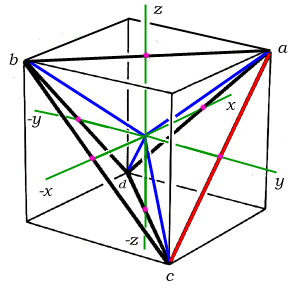More important than what they're called is how they work, and here again we have some divergence in their implementation. QUADRAYS, TETRAYS & SIMPLICIAL COORDINATES The most detailed elaboration of quadrays of which I am personally aware occured on Synergetics-L, subsequent to Chako's getting the ball rolling, and in this context the research forked into quadrays and tetrays as distinct topics, the difference being that tetrays use the identity (1,1,1,1) to tick off an additional, fifth "temporal direction". Possibly we'll end up classifying quadrays as a kind of "simplicial coordinate system", where "simplicial" derives from "simplex", another name for the tetrahedron. The differences in implementation may reduce to conventions around how we choose to normalize our 4-tuples. ALTERNATIVE NORMALIZATIONS Quadrays as originally defined by Chako used only non-negative integers, meaning {a,b,c,0} did not sum to any specific constant k = a+b+c+d. On the other hand, because (d,d,d,d) may be added to (a,b,c,d) with no net effect -- (d,d,d,d)= (0,0,0,0) -- we have the option to normalize to any integer we like. The same rules apply if we allow (a,b,c,d) to be real or floating point numbers. Tom Ace used the k=0 normalization to derive a dot product, and a more streamlined distance method, proving the importance of remaining flexible about how we normalize. ``` def norm0(self): """Normalize such that sum of 4-tuple members = 0""" q = self.quadray() return tuple(map(sub,q,[reduce(add,q)/4.0]*4)) def dot(self,v1): """Return the dot product of self with another vector. return a scalar""" return 0.5 * reduce(add,map(mul,v1.xyz,self.xyz)) def length(self): """Return this vector's length""" return self.dot(self) ** 0.5 ``` MORE QUADRAY METHODS Ace also came up with a cross product method and 4x4 rotation matrices, with (1,0,0,0)... (0,0,0,1) as the axes of rotation, none of which depend on normalization to zero. Here's a cross product method: ``` def cross(self,v1): """Return the cross product of self with another vector. return a Qvector""" A=Qvector((1,0,0,0)) B=Qvector((0,1,0,0)) C=Qvector((0,0,1,0)) D=Qvector((0,0,0,1)) a1,b1,c1,d1 = v1.quadray() a2,b2,c2,d2 = self.quadray() k= (2.0**0.5)/4.0 sum = (A*c1*d2 - A*d1*c2 - A*b1*d2 + A*b1*c2 + A*b2*d1 - A*b2*c1 - B*c1*d2 + B*d1*c2 + b1*C*d2 - b1*D*c2 - b2*C*d1 + b2*D*c1 + a1*B*d2 - a1*B*c2 - a1*C*d2 + a1*D*c2 + a1*b2*C - a1*b2*D - a2*B*d1 + a2*B*c1 + a2*C*d1 - a2*D*c1 - a2*b1*C + a2*b1*D) return k*sum ``` As Tom Ace explains at his website, the above is simply an expansion of the following determinant: ``` A=(1,0,0,0) B=(0,1,0,0) C=(0,0,1,0) D=(0,0,0,1) | A B C D | | k k k k | | a1 b1 c1 d1 | | a2 b2 c2 d2 | ``` VOLUME METHODS Ace also developed a volume method consistent with the usual equivalence in XYZ of a scalar triple product with a determinant i.e. ``` | Ax Ay Ax | A dot (B cross C) = | Bx By Bz | (A,B,C are vectors) | Cx Cy Cz | ``` The scalar triple product likewise gives the volume of a parallelepiped with edges ABC from a common vertex, or of a corresponding tetrahedron, if we multiply the above scalar by k=(1/6) -- plus take the absolute value if we want to eliminate a possible negative sign. The volume of tetrahedron ABCD using quadray coordinates is likewise |k*det[M]| where AB, AC, AD = quadrays (a1,b1,c1,d1),(a2,b2,c2,d2),(a3,b3,c3,d3) and M is the matrix: ``` | 1 1 1 1 | | a1 b1 c1 d1 | | a2 b2 c2 d2 | | a3 b3 c3 d3 | ``` If we set k=(1/4), then the volume of a regular tetrahedron with all edges 1 would be 1 (see below). An regular icosahedron consisting of 20 irregular tetrahedra, with radials of ~0.951 and outer edges = 1, would have a volume of about 18.51. ``` >>> ico = Icosahedron() # create Icosahedron object >>> ico.vertices["O1"].length() # a center-to-vertex vector 0.95105651629515353 >>> ico.volume # compute volume 18.512295868219159 ``` The Icosahedron's constructor method sets the starting volume using the expression: 20*vol1((O1,P1,R1)) where the vol1 method returns (1./4.)*abs(det((O1,P1,R1))). O1,P1,R1 are vectors from the icosa's center to the three vertices of one triangular facet: ```def vol1(qvectors): """Return the volume of a tetrahedron, given 3 quadrays thanks to Tom Ace""" return (1./4.)*abs(det(qvectors)) ``` Vectors define direction. Any 3 non-coplanar edges of a tetrahedron, expressed as vectors, will provide sufficient information to determine the other three edges. These vectors may either share a common vertex, or define an "open triangle" zig-zag. Either way, they provide sufficient input for various vector-based volume methods. In contrast, simple edge lengths, being scalar quantities, do not define direction. To specify three non-coplanar lengths does not uniquely determine the remaining three edges. If we label a tetrahedron's six edges a,b,c,d,e,f -- a,b,c connecting a common vertex to an opposite triangle with edges d,e,f -- then we will need all six values in order to compute a specific volume. Leonhard Euler long ago solved the problem of how to express a tetrahedron's volume solely in terms of its edge lengths. Java programmer Gerald de Jong rederived the method and expressed his result in the form of a Java applet. The computation involves using "closed triangles" (edges from the same face), "open triangles" (3-edge zig-zags), and "opposite pairs" (edges with no endpoints in common). Gerald's expression is also specifically designed to return a volume of 1 when the six edges of the tetrahedron are 1.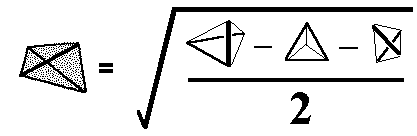Here's a Python version of the algorithm: ```def vol2(edges): """Return the volume of a tetrahedron, 6 edge lengths Thanks to Leonhard Euler and Gerald de Jong """ a2,b2,c2,d2,e2,f2 = map(mul,edges,edges) open = ( f2 * a2 * b2 + d2 * a2 * c2 + a2 * b2 * e2 + c2 * b2 * d2 + e2 * c2 * a2 + f2 * c2 * b2 + e2 * d2 * a2 + b2 * d2 * f2 + b2 * e2 * f2 + d2 * e2 * c2 + a2 * f2 * e2 + d2 * f2 * c2 ) closed = ( a2 * b2 * d2 + d2 * e2 * f2 + b2 * c2 * e2 + a2 * c2 * f2 ) oppos = ( a2 * e2 * (a2 + e2) + b2 * f2 * (b2 + f2) + c2 * d2 * (c2 + d2)) return (abs(open - closed - oppos)/2.0)**0.5 ``` This method has nothing specifically to do with quadrays, since length is an attribute of the generic Vector class. I mention it more because of its historical role in the quadray thread on Synergetics-L. David Chako conjectured that all tetrahedra with vertices at the centers of fcc spheres might have whole number volumes, provided the initial tetrahedron -- defined by four intertangent fcc spheres -- were defined as volumetric unity. Until Robert Gray developed an algebraic proof for this conjecture, de Jong's Java applet and a MathCad worksheet featuring Euler's original formula, provided an initial source of empirical evidence for its validity (i.e. failed to turn up any counter-examples). Other volume methods would have served, but this was how events actually unfolded. In terms of quadrays, fcc sphere centers may be expressed as sums of vectors with coordinates {2,1,1,0}. For example, if s were an fcc sphere center, (2,1,1,0) + (1,1,2,0) + (1,2,0,1) = (4,4,3,1) = (3,3,2,0) would be another fcc sphere center relative to s. I will return to quadrays in a sphere packing context in the notes on crystallography below.
 CONVERTING TO/FROM XYZ (ONE POSSIBLE IMPLEMENTATION) Differences in implementation may arise around how we choose to define quadrays in relation to XYZ. Perhaps the most obvious convention would be to define the length of each basis vector {1,0,0,0} as unity. However, in my own research, it's more useful and practical to apply a different "control length", such that the six edges of the "home base" tetrahedron are either 1 or 2 units. This is because my home base tetrahedron is constructed by closest- packing four equi-radius spheres, such that sphere centers define the tetrahedron's four vertices. This means the tetrahedron's edges are 2R (R=sphere radius) or 1D (1=sphere diameter). Therefore I want my four quadrays to have a length of root(6)/2 or root(6)/4 respectively.``` >>> v = Qvector((1,0,0,0)) >>> v.length() 0.61237243569579447 >>> 6**0.5/4.0 # compare with root(6)/4 0.61237243569579447 ``` With these preliminaries out of the way, we have sufficient information to uniquely pair normalized (a,b,c,d) Qvectors with Cartesian (x,y,z) Vectors and vice versa: From the Vector class: ``` def quadray(self): """return (a,b,c,d) quadray based on current (x,y,z)""" x=self.xyz y=self.xyz z=self.xyz a = (2/root2) * ((x>=0)*x + (y>=0)*y + (z>=0)*z) b = (2/root2) * ((x<0)*(-x) + (y<0)*(-y) + (z>=0)*z) c = (2/root2) * ((x<0)*(-x) + (y>=0)*y + (z<0)*(-z)) d = (2/root2) * ((x>=0)*x + (y<0)*(-y) + (z<0)*(-z)) return self.norm((a,b,c,d)) ``` From the Qvector subclass of Vector: ``` def __init__(self,arg,*flag): """Initialize a vector at an (a,b,c,d) tuple (= arg). NOTE: in accompanying essay, xyz units = sphere diameter i.e. Vector((1,0,0)).length() is 1 D, therefore quadray inputs must be scaled by 1/2 to fit this context, i.e. tetra edge defined by 2 basis quadrays = 1 D.""" if len(arg)==3: arg = Vector(arg).quadray() # if 3-tuple passed self.coords = self.norm(arg) a,b,c,d = self.coords self.xyz = ((0.5/root2) * (a - b - c + d), (0.5/root2) * (a - b + c - d), (0.5/root2) * (a + b - c - d)) ``` Let's look at these methods in action: ``` >>> from coords import * >>> i = Vector((1,0,0)) >>> i.xyz (1, 0, 0) >>> i.quadray() (1.4142135623730949, 0.0, 0.0, 1.4142135623730949) >>> j = Vector((-1,0,-3)) >>> q = Qvector(j.quadray()) >>> q Qvector (0.0, 1.4142135623730949, 5.6568542494923797, 4.2426406871192848) >>> q.xyz (-0.99999999999999978, 0.0, -2.9999999999999996) ```
 Note that floating point numbers lose a small amount of information as we convert back and forth between Qvectors and Cartesian Vectors (or "Cvectors"). USEFULNESS IN SPATIAL GEOMETRY "Are quadrays useful?", is the final question I should address, as I reach the close of this essay. I've found them to be so in the context of conceptualizing the relationships among polyhedra. The initial home base tetrahedron with coordinates (1,0,0,0), (0,1,0,0), (0,0,1,0), (0,0,0,1) will generate 22 other points of interest by simple vector addition (negation is not required). These 26 points A-Z are sufficient to define a tetrahedron, inverse tetrahedron, cube, octahedron, rhombic dodecahedron and cuboctahedron (David Chako supplied coordinates for the first few of these in his initial post on the topic). Furthermore, the size and orientation of these six shapes matches that given by R. Buckminster Fuller in his Synergetics, wherein these shapes all have whole number volumes, given the tetrahedron is Fuller's geometric model of 3rd powering (i.e. 1x1x1 = unit volume tetrahedron).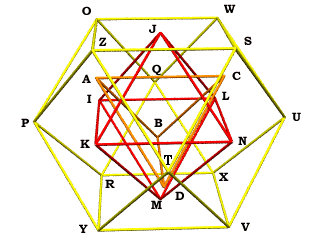So with Qvectors, we bring whole number coordinates to an initial set of shapes which already have whole number volumes. This is an aesthetically pleasing construct, and may help streamline the introduction to both spatial geometry and object-oriented programming, as per my "numeracy + computer literacy" approach (currently Python-focused).
 ```""" By Kirby Urner, Oregon Curriculum Network Ver 0.2: May 29, 2000 """ from coords import * from operator import mul import math #==================[ Points of Interest ]==================== """ * 26 data points A-Z define six polyhedra in the concentric hierarchy Labels of Numbers of Shape Volume Vertices Vertices, Edges, Faces ------------------------------------------------------------- Tetrahedron 1 A -D 4 6 4 Inv Tetra 1 E -H 4 6 4 Duo-tet Cube 3 A -H 8 12 6 Octahedron 4 I -N 6 12 8 Rh Dodecahedron 6 A -N 14 24 12 Cuboctahedron 20 O -Z 12 24 14 See: http://www.4dsolutions.net/ocn/graphics/povlabels.gif Using the quadray apparatus (4 basis vectors to the corners of a regular tetrahedron with edges = 1 sphere diameter) See: http://www.grunch.net/synergetics/quadray/quadray.gif """ A = Qvector((1,0,0,0)) # center to corner of tetrahedron B = Qvector((0,1,0,0)) # " C = Qvector((0,0,1,0)) # " D = Qvector((0,0,0,1)) # " # tetrahedron's dual (also a tetrahedron i.e. inverted tet) E,F,G,H = B+C+D,A+C+D,A+B+D,A+B+C # tetrahedron + dual (inverted tet) = duo-tet cube # octahedron vertices from pairs of tetrahedron radials I,J,K,L,M,N = A+B, A+C, A+D, B+C, B+D, C+D # octahedron + dual (cube) = rhombic dodecahedron # cuboctahedron vertices from pairs of octahedron radials O,P,Q,R,S,T = I+J, I+K, I+L, I+M, N+J, N+K U,V,W,X,Y,Z = N+L, N+M, J+L, L+M, M+K, K+J ... ```
 APPLICATIONS TO CRYSTALLOGRAPHY Quadrays take to a sphere packing context like fish to water and so might serve some streamlining role in crystallography. Scott Childs has researched this possiblity. Russell Chu's investigations are also relevant in this connection. The 12 quadrays {2,1,1,0} define the vertices of a cuboctahedron relative to the origin (0,0,0,0). This arrangement, with 12 nearest neighbors at the corners of a cuboctahedron, is the face centered cubic lattice (fcc). Quadrays with integer coordinates define the centers of fcc spheres, as well as the centers of all tetrahedral and octahedral voids between these spheres. Given the volume expression (1/4)|det[M]|, we know that any non-coplanar arrangement of any four such vertices (i.e. with integer coordinates) will define a tetrahedral volume evenly divisible by 1/4. Those tetrahedra with all vertices in the same fcc lattice will have whole number volumes (a sufficient, but not a necessary condition). Selectively illuminated, these all-integer, quadray defined vertices may present face centered, body centered, hexagonally close packed, or simple cubic arrangements of points. We may also sort all these vertices into four interpenetrating fcc lattices, using the rhombic dodecahedron for guidance. Rhombic dodecahedra fill space in an fcc pattern, with the spheres inscribed and intertangent through the rhombic face centers. The corners of the rhombic dodecahedron occur at the centers of the voids surrounding each fcc sphere.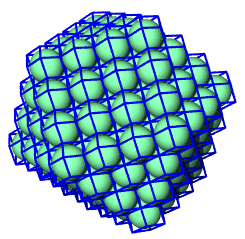The rhombic dodecahedron has 8 points at the opposite ends of short face diagonals, in a cubic arrangement -- this is the cube of volume 3, relative to the dodeca's volume of 6. This cube defines two interpenetrating unit-volume tetrahedra, A-D and E-H, as per the above labeling scheme (see 26 points of interest). To each tetrahedron, there corresponds a unique fcc lattice. In addition, the remaining 6 vertices of the rhombic dodecahedron, which occur at opposite ends of long face diagonals, define an octahedral arrangement. The corresponding vectors, I-N, define yet another fcc lattice.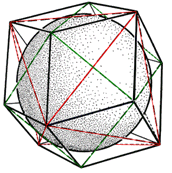The sphere at the center of our rhombic dodecahedron, at (0,0,0,0), has 12 nearest neighbors O-Z. This is the original lattice. So we may identify the four lattices as follows: {(1:'O-Z'),(2:'I-N'),(3:'A-D'),(4:'E-H')}. Vertices from 1+2, or 3+4, will define a simple cubic lattice. All 4 lattices taken together will define a body cubic centered lattice. The hcp arrangement cannot be expressed as a sum of whole fcc lattices, but its vertices will nevertheless have all integer quadray coordinates. Background Reading: An Introduction to Quadrays (lots of pictures, uses tetra edge = 2R instead of 1D) Numeracy + Computer Literacy essay (a math-through-programming approach) Python source code (HTML) and text only versions. Return to Relevant Resources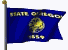Oregon Curriculum Network